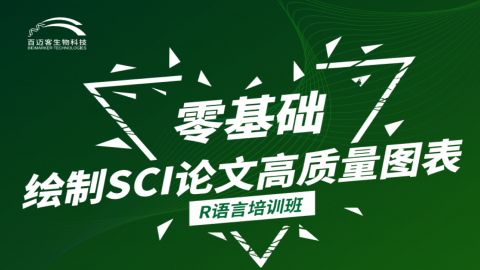R语言绘图基础259人加入学习
(1人评价)

[展开全文]

[展开全文]

1.数值型：一串数字，可单独存在；字符型：一个字母或单词，必须用双引号或单引号括起来

[展开全文]

[展开全文]

seq(from = 1, to = 10, by = 1)

seq( from = 1, to = 10, length.out = 4)

seq(from =1, to = 10, length.out = 5)

BMK <- c('B','M','K')

seq(along.with = BMK)

rep() 重复次数

times 复制多少次 ，正数

each 每次

length.out 返回向量的长度

BMK <- c('B','M','K')

mode(BMK)

length(BMK)

rep(x = BMK,times = 2)

rep(x = BMK,each = 2)对象中每个元素复制2次

rep(x= BMK, each = 2, length.out = 4)

sort()

BMK <- c(2,5,4,1)

sort(BMK)

sort(BMK, decreasing = T)

sort(BMK, decreasing = F)

BMK <- c(2,5,4,1)

BMK

rev(BMK)反转

BMK <-c(2,2,1,3)

unique(BMK)向量去除

which() 条件语句

== > < >= <= !=

2!= 2

BMK <-c(“B”,"M", "K")

which是真实的索引

[展开全文]

[展开全文]

Biocloud